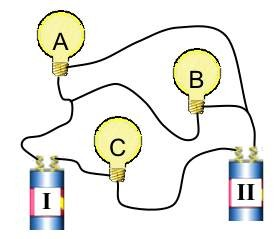Teaching Physics with the Physics Suite Edward F. Redish Problems Sorted by Type | Problems Sorted by Subject | Problems Sorted by Chapter in UP

Three bulbs and two batteries

 In the figure at the right is shown a circuit with two identical batteries and three identical resistors (bulbs). The batteries are shown so that the anode (positive terminal) is on the right of each battery. If one of these batteries were to be directly connected to one of these bulbs, it would light the bulb brightly. For the following items, select the item that best completes the sentence and enter it in your answer sheet. If more than one answer is correct, give them all. Briefly explain your answers.A. When the set of batteries and bulbs are connected as shown

1. none of the bulbs will be lit.
2. only one of the bulbs will be lit.
3. all of the bulbs will be lit equally brightly.
4. all of the bulbs will be lit but they will not be equally bright.
5. you cannot tell which bulbs will be lit from the information given.

B. The current through bulb C

1. equals zero.
2. is the same as through the other two bulbs.
3. is greater than through either of the other bulbs.
4. is less than through either of the other bulbs.
5. is the same as the current in battery I.
6. is the same as the current in battery II.
7. cannot be determined from the information given.

C. The potential drop across bulb B

1. equals zero.
2. is the same as that across bulb A.
3. is the same as that across bulb C.
4. is the same as that across one of the batteries.
5. is double that across one of the batteries.
6. cannot be determined from the information given.